• 一、知识目标
• 二、知识点
• 01、生成器
• ①、生成器表达式
• ②、yield函数
• 02、迭代器
• ①、iter函数
• ②、next函数
• ③、自定义迭代器

• # 一、知识目标

1、掌握可迭代对象、迭代器与生成器的概念
2、理解可迭代对象的分类方法
3、掌握迭代器与生成器的使用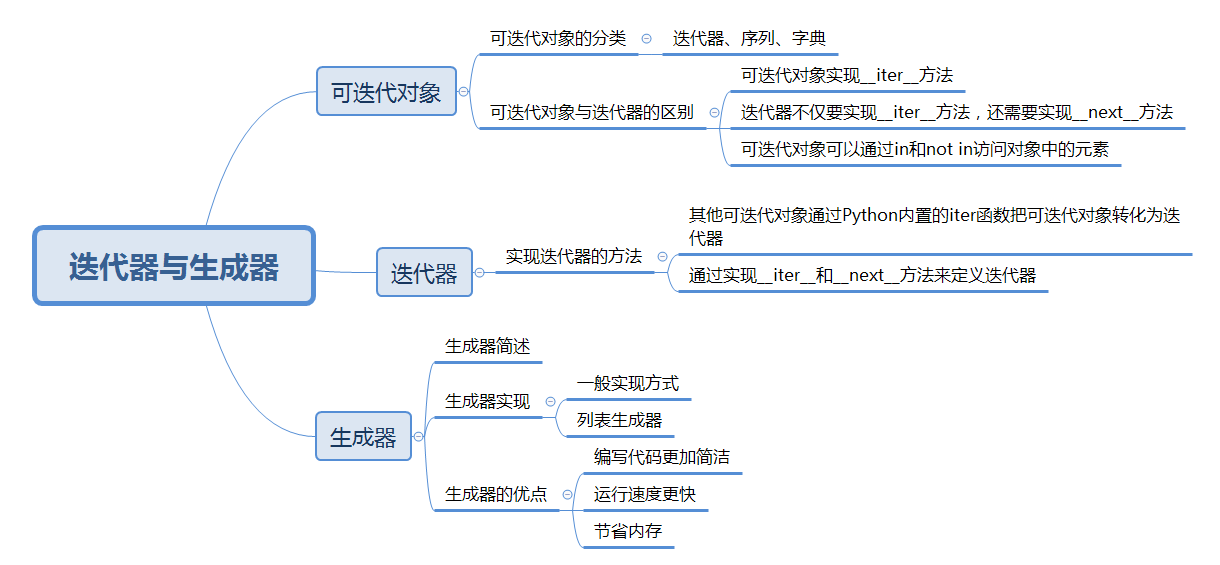# 二、知识点

## 01、生成器

### ②、yield函数

~~在python中使用了yield的函数被称为生成器（generator）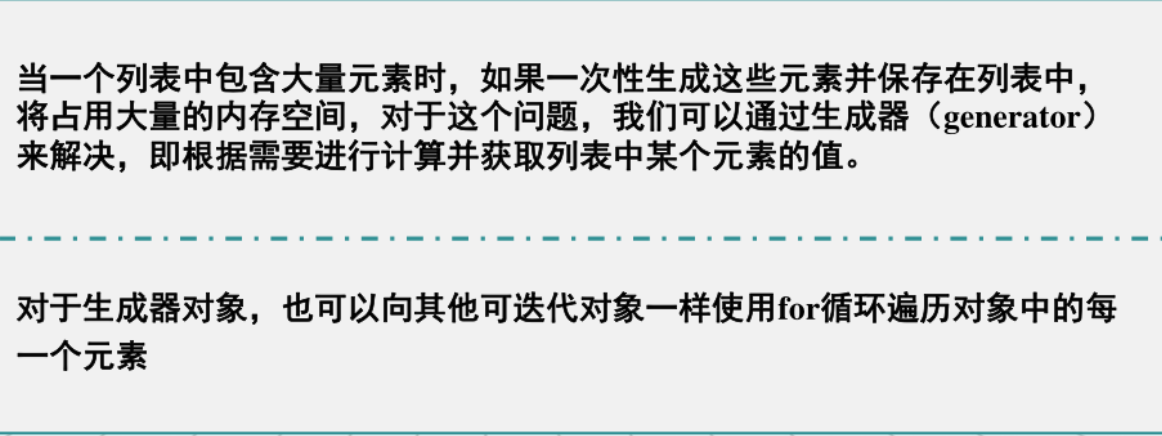~~将列表生成表达式中的一对中括号改为小括号即可得到生成器。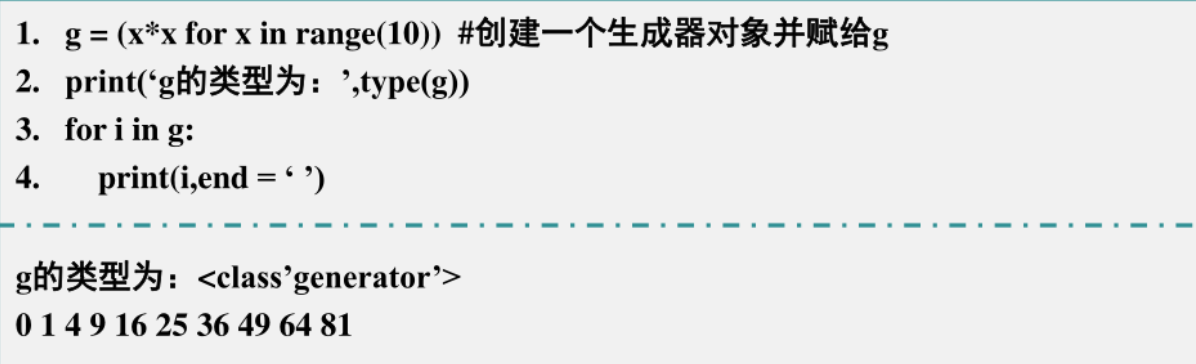~~如果生成元素的方法比较复杂，不适合用for循环方式实现，我们还可以借助yield关键字利用函数实现生成器的功能。例子：实现faclist函数，依次生成1的阶乘、2的阶乘、、、、n的阶乘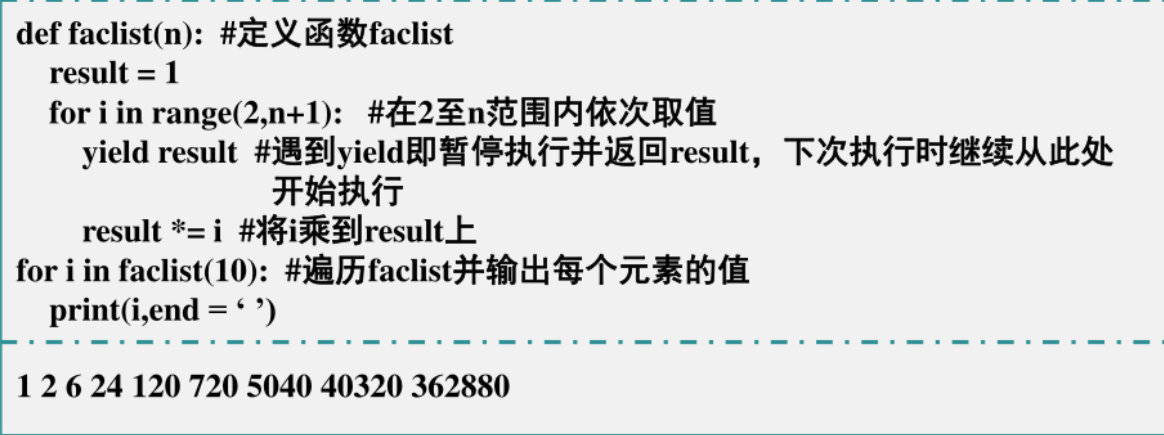使用yield实现斐波那契数列：

``````import sys

def fibonacci(n):  # 生成器函数-斐波那契
a, b, counter = 0, 1, 0
while True:
if (counter > n):
return n
yield a
a, b = b, a + b
counter += 1

f = fibonacci(10)  # f是一个迭代器，由生成器返回生成
while True:
try:
# ",".join(next(f))  # 空暇在尝试，，此处有一个小bug
print(next(f), end=",")
except StopIteration:
sys.exit()
``````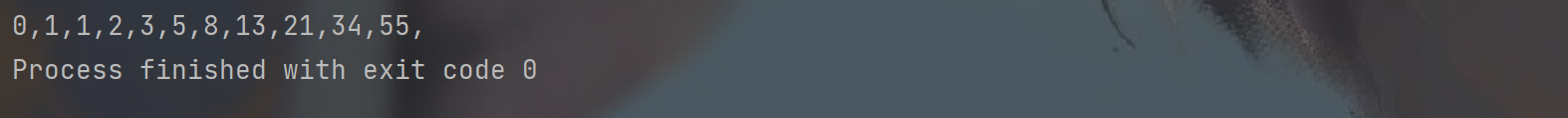## 02、迭代器

### ①、iter函数

迭代器（Iterator）是指可以通过next函数不断获取下一个值的对象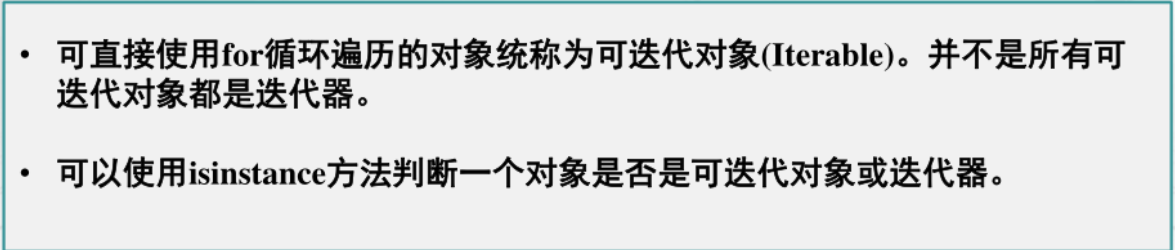例如：

``````# 例子：字符串、列表或元组都可以用于创建迭代器
list = [1, 2, 3]
it = iter(list)  # 创建迭代器对象
print(next(it))  # 输出迭代器的下一个元素
print(next(it))
# for i in it:
#     print(i,end="")

# 判断可迭代对象和迭代器
from collections.abc import Iterator, Iterable

ls = [1, 2, 3, 4, 5]  # 创建一个列表对象
g = (x for x in range(1, 6))  # 创建一个生成器
print("ls是可迭代对象:", isinstance(ls, Iterable))
print("g是可迭代对象:", isinstance(ls, Iterable))
print("ls是迭代器:", isinstance(ls, Iterator))
print("g是迭代器:", isinstance(ls, Iterator))

``````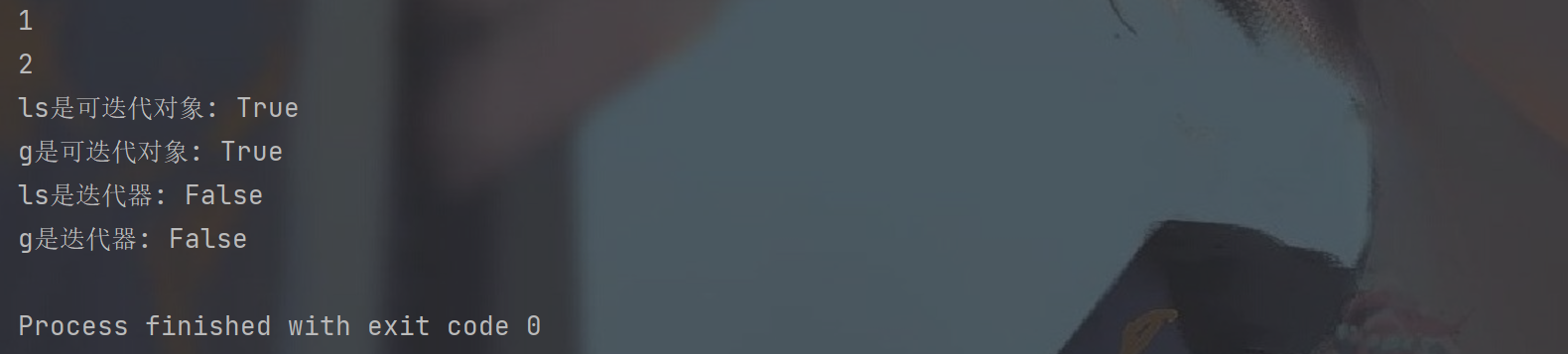~~iter函数：对于可迭代对象，可以通过iter函数得到迭代器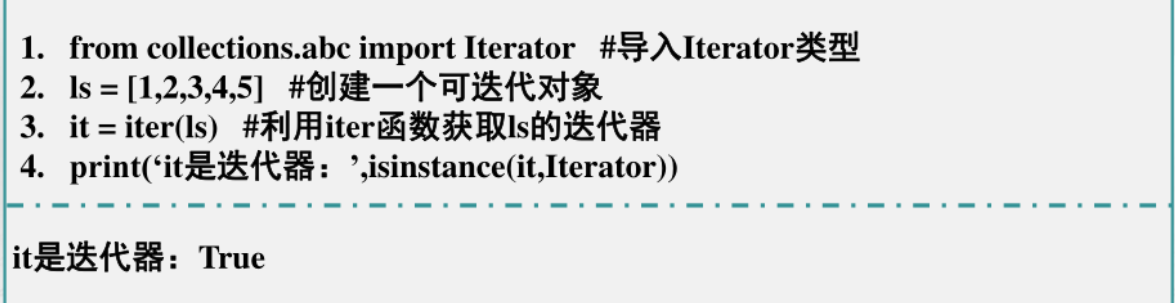### ②、next函数

~~对于迭代器，则可以使用next函数不断获取下一个元素，当所有元素都获取完毕后在调取next函数，就会引发StopIteration。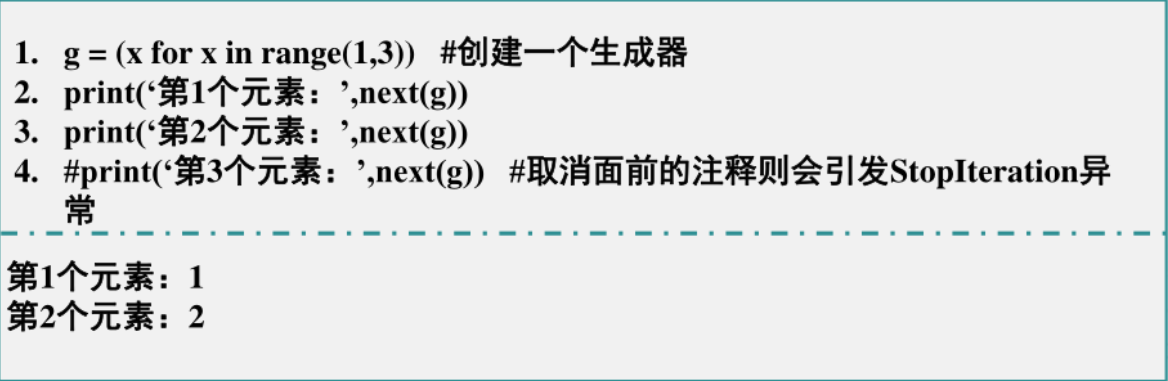~~处理StopIteration异常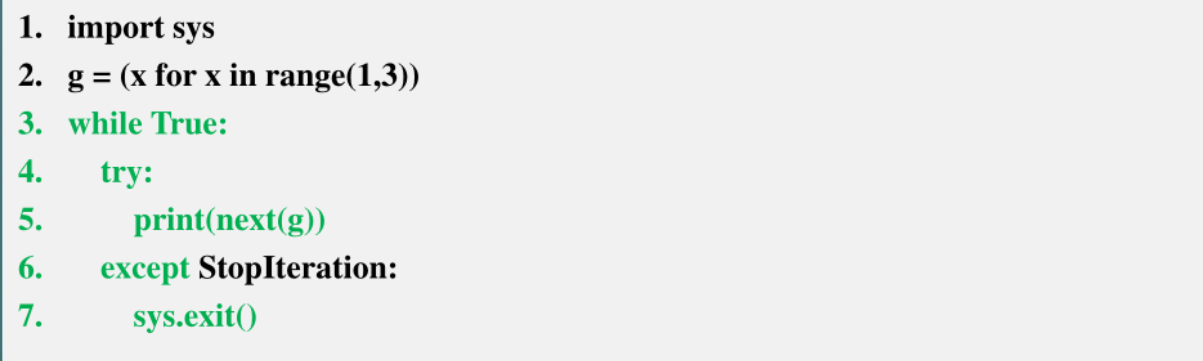### ③、自定义迭代器

使用__next__和__iter__

``````from collections.abc import Iterator

class Faclist:  # 定义Faclist类
def __init__(self):  # 定义构造方法
self.n = 1
self.fac = 1

def __next__(self):  # 定义next方法
self.fac *= self.n
self.n += 1
return self.fac

def __iter__(self):  # 定义iter方法
return self

if __name__ == '__main__':
facs = Faclist()
print('facs是迭代器：', isinstance(facs, Iterator))
for i in range(1, 6):  # i在1至5范围依次取值
print('第%d个元素：' % i, next(facs))

``````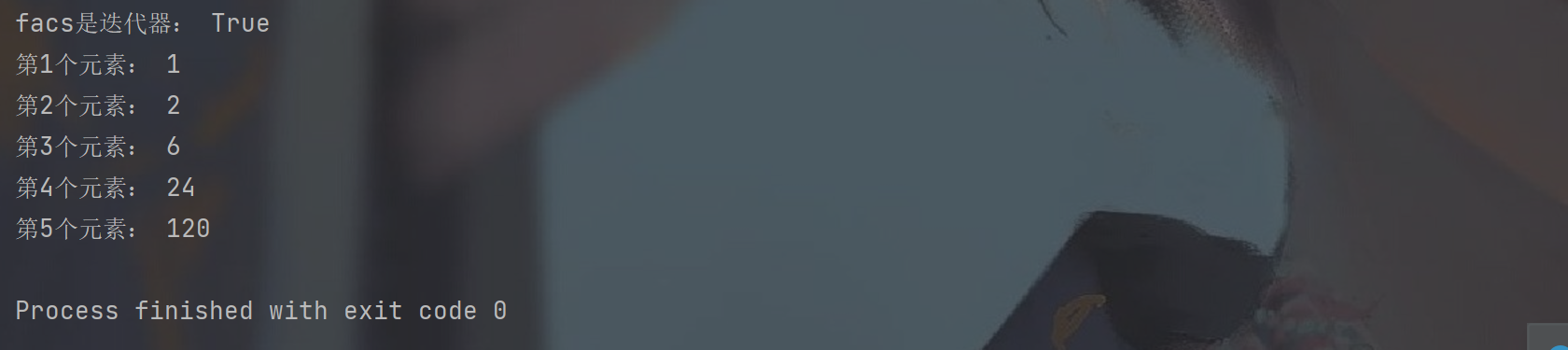来源：Argonaut_